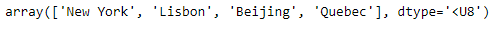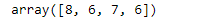Open in App
Not now

# Find the length of each string element in the Numpy array

• Last Updated : 02 Jan, 2023

NumPy builds on (and is a successor to) the successful Numeric array object. Its goal is to create the corner-stone for a useful environment for scientific computing. NumPy provides two fundamental objects: an N-dimensional array object (ndarray) and a universal function object (ufunc). In this post we are going to discuss ways in which we can find the length of each string element in the given numpy array. Problem #1 : Given a numpy array whose underlying data is of string type. Find the length of each element in the given object. Solution : We are going to use numpy.vectorize() function to find the length of each element in the given numpy array object.

## Python3

 `# importing the numpy library as np``import` `numpy as np` `# Create a numpy array``arr ``=` `np.array([``'New York'``, ``'Lisbon'``, ``'Beijing'``, ``'Quebec'``])` `# Print the array``print``(arr)`

Output :Now we will use numpy.vectorize() function to find the length of each element in the given numpy array object.

## Python3

 `# Use vectorize function of numpy``length_checker ``=` `np.vectorize(``len``)` `# Find the length of each element``arr_len ``=` `length_checker(arr)` `# Print the length of each element``print``(arr_len)`

Output :As we can see in the output, we have successfully calculated the length of each string element in the given numpy array object. Problem #2 : Given a numpy array whose underlying data is of string type. Find the length of each element in the given object. Solution : We are going to use List comprehension technique to find the length of each element in the given numpy array object.

## Python3

 `# importing the numpy library as np``import` `numpy as np` `# Create a numpy array``arr ``=` `np.array([``'New York'``, ``'Lisbon'``, ``'Beijing'``, ``'Quebec'``])` `# Print the array``print``(arr)`

Output :Now we will use List comprehension technique to find the length of each element in the given numpy array object.

## Python3

 `# Find the length of each element``arr_len ``=` `[``len``(i) ``for` `i ``in` `arr]` `# Print the length of each element``print``(arr_len)`

Output :As we can see in the output, we have successfully calculated the length of each string element in the given numpy array object.

One additional approach to finding the length of each element in a NumPy array is to use the NumPy function numpy.char.str_len. This function takes a NumPy array of strings as input and returns an array of the lengths of each string. For example:

## Python3

 `import` `numpy as np` `# Create a numpy array``arr ``=` `np.array([``'New York'``, ``'Lisbon'``, ``'Beijing'``, ``'Quebec'``])` `# Find the length of each element``arr_len ``=` `np.char.str_len(arr)` `# Print the length of each element``print``(arr_len)` `#This code is contributed by Edula Vinay Kumar Reddy`

This will output: [8 7 7 6] (This code wont work in gfg compiler , install numpy in your local compiler then check )

My Personal Notes arrow_drop_up